# Example Toolboxes 2D building

$\quad$In this example, we will build a thermal model of the building. The strategy is to complicate the simple 2D model by adding physical parameters and using equivalent approximation terms and numerical methods of finite element. The Toolboxe `heat` will be used to solve the problem.

$\quad$The construction of the model requires a well-posed problem, that is to say, if from a theoretical point of view the equations have many unique solutions. For this, we need to make the weak formulations of the equations we will use. This is a part that remains important, because if the problem is poorly posed, then we may have no solution or several. This gives false numerical schemes and the simulations made may be wrong.

## 1. Running the case

The command line to run this case is

``mpirun -np 8 feelpp_toolbox_heat --case "github:{repo:toolbox,path:examples/modules/heat/examples/2Dbuilding}"``

## 2. Data files

The case data files are available in Github here

## 3. 2D thermal model

$\quad$The 2D thermal model of two parts separated by plaster is considered. It is an object of $\mathbb {R} ^ 2$, it is subdivided into different domains $\Omega_i, i = 1,2,3$ (see the image). The sources of heat $heater_i, i = 1,2$ are the two radiators each of which is located downstairs in each room. The heat $T$ released in the subdomains is calculated thanks to the equation of heat transfer.

We use model multi-physique of heat tranfert with $k_i (y)$ which is the thermal conductivity which depends the coordinate from the stem height:[y], and we have $0 \leq y \leq H$.

$k_i(y) = k_i^{ground} + \frac{y}{H} \left( k_i^{roof} - k_i^{ground}\right)$

$\ quad$ We notice that in this first model, taking the parameter $k_i (y)$ increasing linear, varied according to the height, we can simulate the effect that the cold air is at the bottom and the warm air diffuses towards the ceiling. This is the effect of natural convection. This is an improvement of the model with $k$ constant.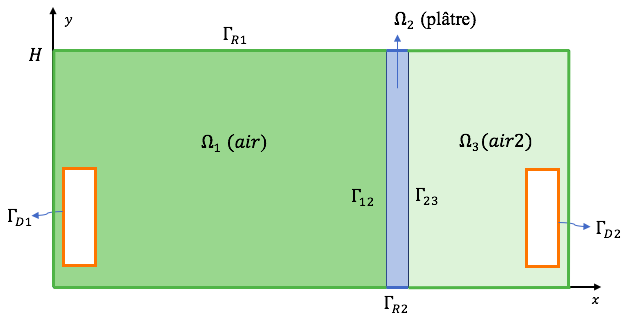The conditions at the edges:

• Condition of Dirichlet

$T = heater_i \quad \text{in} \quad \Gamma_{Di}$
• Condition of Robin

$- k_i(y) \nabla T \cdot n = h_i (T -T_{ext}) \quad \text{in} \quad \Gamma_{Ri} \\$

Conditions at the interface $\Gamma_{ij} = \Omega_i \cap \Omega_j$

$T_i = T_j \\ k_i \nabla T \cdot n_i = - k_j \nabla T \cdot n_j$

Initial condition to $t = 0s$

$T = T_0$

### 3.1. Input

Table 2. Parameter table
Notation Description Value Unit Note

Paramètres globale

$t$

times

s

$T$

temperature

$K$

$T_{ext}$

outside temperature

280

$K$

$T_0$

initial temperature

280

$K$

$H$

height

$1,2,3$

$m$

Air

$k_1(y)$

thermal conductivity

$W.m^{-3}.K^{-1}$

$k_1^{ground}$

k_1(0)

$1.,1.5.,2.$

$W.m^{-3}.K^{-1}$

$k_1^{roof}$

k_1(H)

$4.,5.,6.$

$W.m^{-3}.K^{-1}$

$\rho_1$

mass volumique

1

$kg/(m^3)$

$C_1$

thermal capacity

1004

$J/(kg*K)$

$h_1$

heat transfer coefficient

1.0/(0.06+0.01/0.5 + 0.3/0.8 + 0.20/0.032 +0.016/0.313 +0.14)

$W.m^{−2}.K^{−1}$

$heater_1$

heat source

310

$K$

Air2

$k_2(y)$

thermal conductivity

$W.m^{-3}.K^{-1}$

$k_2^{ground}$

k_2(0)

$1.,1.5.,2.$

$W.m^{-3}.K^{-1}$

$k_2^{roof}$

k_2(H)

$4.,5.,6.$

$W.m^{-3}.K^{-1}$

$\rho_2$

mass volumique

1

$kg/(m^3)$

$C_2$

thermal capacity

1004

$J/(kg*K)$

$h_2$

heat transfer coefficient

1.0/(0.06+0.01/0.5 + 0.3/0.8 + 0.20/0.032 +0.016/0.313 +0.14)

$W.m^{−2}.K^{−1}$

$heater_2$

heat source

300

$K$

Plâtre

$k_3(y)$

thermal conductivity

$W.m^{-3}.K^{-1}$

$k_3^{ground}$

k_3(0)

$0.25$

$W.m^{-3}.K^{-1}$

$k_3^{roof}$

k_3(H)

$0.25$

$W.m^{-3}.K^{-1}$

$\rho_3$

mass volumique

150

$kg/(m^3)$

$C_3$

thermal capacity

1000

$J/(kg*K)$

$h_3$

heat transfer coefficient

1.0/(0.06+0.01/0.5 + 0.3/0.8 + 0.20/0.032 +0.016/0.313 +0.14)

$W.m^{−2}.K^{−1}$

### 3.2. Numerical experiences

#### 3.2.1. Geometry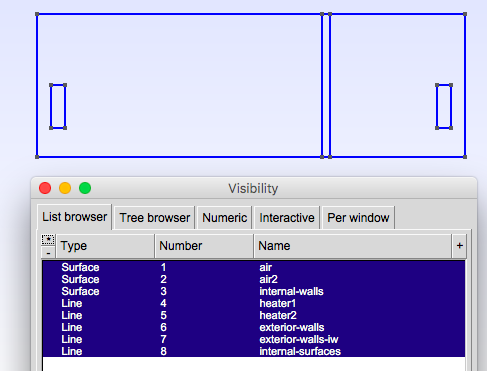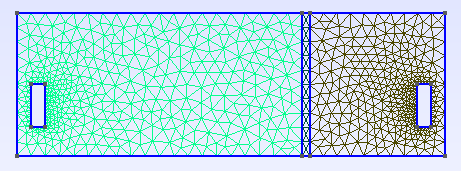Parameter to vary
``````h=0.1;
hHeater=h/5.;

W=2; //height
w=1; //width
ep1=0.1; //plaster width``````

## 4. Implementation

Note on the definition of the function $k_i(y)$ in the `.cfg` file

``````directory=applications/ibat/heat-transfert/toolbox
case.dimension=2``````

mesh.filename=$cfgdir/thermo2d.geo gmsh.hsize=0.01#0.05 filename=$cfgdir/thermo2d.json

initial-solution.temperature=280

#do_export_all=true #verbose=1 #verbose_solvertimer=1 reuse-prec=1 pc-type=gamg

order=2

time-step=300 time-final=5e4 restart.at-last-save=true

``````{
"Name": "Thermo dynamics",
"ShortName":"ThermoDyn",
//"Model":"Thermic",
"Parameters":
{
"kground":"0.001",
"kroof":"2.9"
},
"Materials":
{
"air":
{
"markers":"air",
//"k":"2.9",//[0.0262 W/(m*K) ]
"rho":"1",
"mu":"2.65e-2",
"k":"kground+ (y^6)*(kroof-kground):y:kroof:kground",
"Cp":"1004"
},
"air2":
{
"markers":"air2",
//"k":"2.9",//0.0262[ W/(m*K) ]
"rho":"1",
"mu":"2.65e-2",
"k":"kground+ (y^6)*(kroof-kground):y:kroof:kground",
"Cp":"1004"
},
"internal-walls":
{
"markers":"internal-walls",
"k":"0.25",//[ W/(m*K) ]
//"k11":"0.0262"//[ W/(m*K) ]
"Cp":"1000", //[ J/(kg*K) ]
"rho":"150" //[ kg/(m^3) ]
}
},
"BoundaryConditions":
{
"temperature":
{
"Dirichlet":
{
"heater1": { "expr":"310"/*"330"*/ },
"heater2": { "expr":"310"/*"320"*/ }
},
"Robin":
{
"exterior-walls":
{
"expr1":"1.0/(0.06+0.01/0.5 + 0.3/0.8 + 0.20/0.032 +0.016/0.313 +0.14)",// h coeff
"expr2":"280"// temperature exterior
},
"exterior-walls-iw":
{
"expr1":"1.0/(0.06+0.01/0.5 + 0.3/0.8 + 0.20/0.032 +0.016/0.313 +0.14)",// h coeff
"expr2":"280"// temperature exterior
}
}
}
},
"PostProcess":
{
"Exports":
{
"fields":["temperature","pid"]
}
}``````

}

Command line to execute
``mpirun -np 16 feelpp_toolbox_heat_2d --config-file thermo2d.cfg``
Command line to execute using the `case` with link to folder which represents a remote data in a github repository.
``mpirun -np 8 feelpp_toolbox_heat --case "github:{repo:toolbox,path:examples/modules/heat/examples/2Dbuilding}"``

You can also use some options to change the variables like `time-final`, taille du maillage `hsize`, …​

``mpirun -np 8 feelpp_toolbox_heat --case "github:{repo:toolbox,path:examples/modules/heat/examples/2Dbuilding}" --ts.time-final 3000``

## 5. Result

Choose k following an equivalent value $k_{eq} = 2.9$ (see ) then generate the value of $k^{ground}$ and $k^{roof}$ follow a normal distribution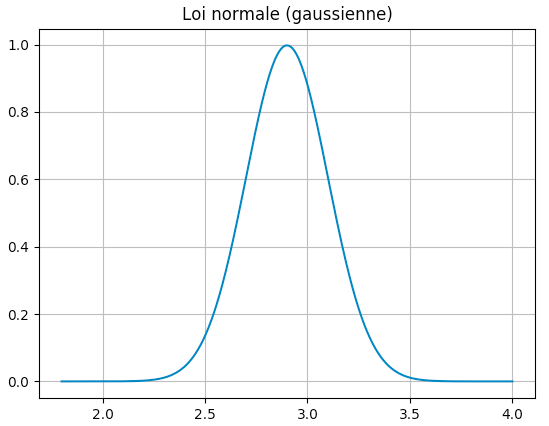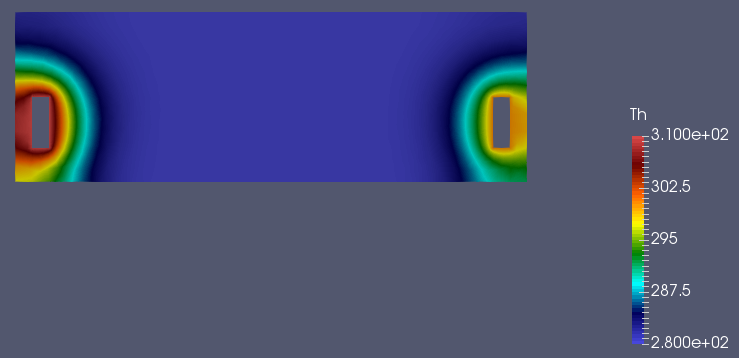Utilise la fonction $k(y)$ non linéaire

$k_i(y) = k_i^{ground} + \left( k_i^{roof} - k_i^{ground} \right) y^4$
 $k^{ground} = 0. , k^{roof} = 2.9 , tmax=1000, dt = 0.01$ $k^{ground} = 0. , k^{roof} = 2.9 , tmax=150000, dt = 500$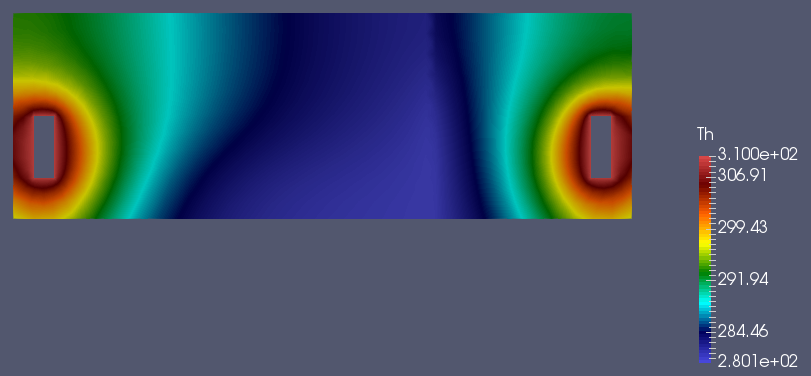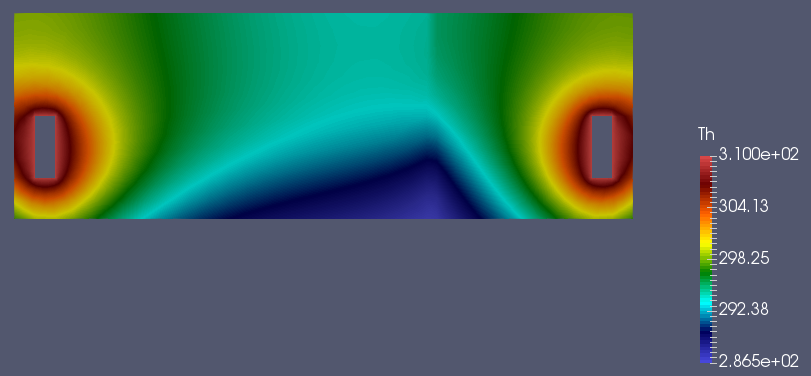Uses the function $k(y)$ nonlinear with powerful 6

$k_i(y) = k_i^{ground} + \left( k_i^{roof} - k_i^{ground} \right) y^6$
 $k^{ground} = 0. , k^{roof} = 2.9 , tmax=1000$ $k^{ground} = 0. , k^{roof} = 2.9 , tmax=1000, dt=300$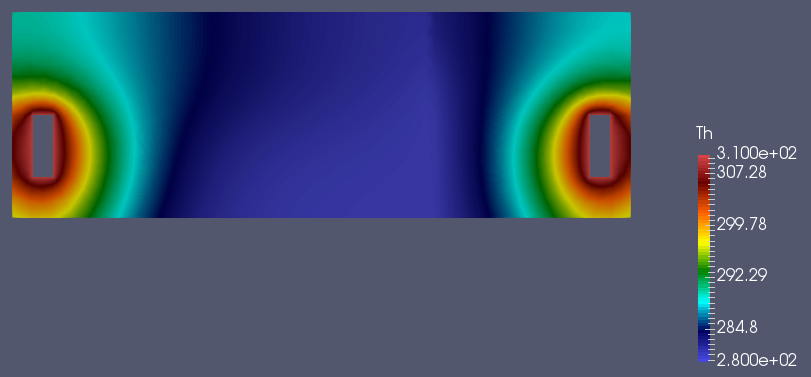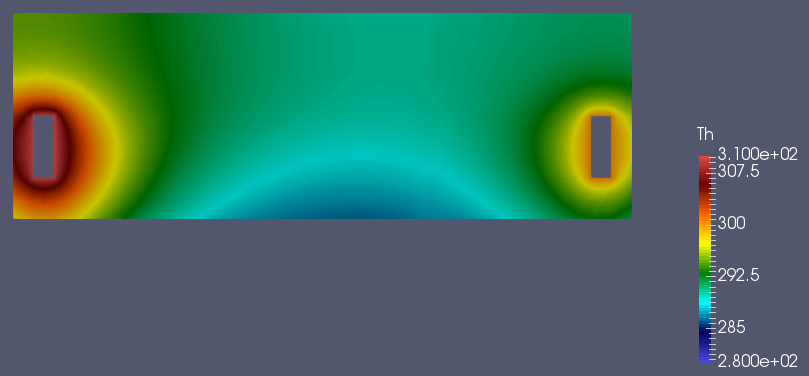## 6. Biblio

 A.W.M (Jos) van Schijndel * BES with FEM: Building Energy Simulation using Finite Element Methods *